## Electrical signal power and energy calculations by example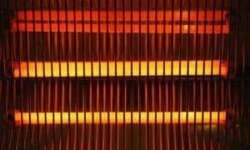In electronics and signal processing, you have to deal with electrical signals. In many cases, you may need to calculate signal power and energy. Power and energy for DC In a standard situation, when DC power supply is applied to a known resistor or another device like an LED motor you can calculate its power very easy by applying Ohms law: If we run this device for time T then we can calculate total energy used: In some cases, you may not know the resistance of your circuit. In this case, you can measure the current flow. So your power formula can be transformed by using same Ohms law:

## Using wavelet transform in biomedical engineering – heart signal analysis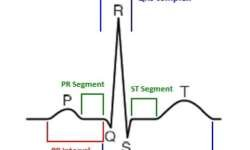In previous post, we cleared out that wavelet transform is used to analyze short-time and non-stationary signals. Since base wavelet function has to parameters – translation and scaling, it is possible to achieve good time and frequency localization. In other words, we can equally analyze the slow signal and fast signal structures without losing resolution and so evaluate signal frequency characteristics and time dynamics. Heart signal analysis is one of the most common problems in biomedical engineering. Practically every part of ECG signal carries some sort of information about heart conditions, possible pathologies, and diseases. So equally, frequency and timing characteristics of ECG signal is essential. As you know standard ECG signal consists of several typical waveforms like P-QRS-T, where in P and T waves low frequency component dominates, and in QRS, mid and high. The common condition of hear is myocardial ischemia when blood flow through coronary arteries to the heart is reduced, what prevents receiving enough oxygen. This can damage the heart muscle and lead to a heart attack. In order to notice this pathology it is we need to analyze S-T segment of ECG waveform. Insignificant changes in the signal can indicate ischemia. In order to find…

## Wavelet transform – the basics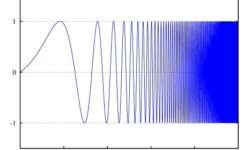Recently I’ve been reviewing wavelet transform. I think some points are worth sharing. In this first post, I would like to mention the basics of wavelet transform and its main features. A probably most important question that comes to mind is why we need another transform when we already have Fourier transform. The answer lies in the signals that we want to analyze. If we take any periodic signal that is stationary (e.g., Pulse train) then Fourier transform is the right tool because its frequency components don’t change in time. Let’s take another case when the signal changes in time (chirp signal). Classical Fourier transform cannot determine frequency components of such signal because it doesn’t carry any information about signal time scale. Of course, it is possible to cheat with Short Time Fourier Transform (STFT) when the signal is analyzed in short chunks, but again there is a problem of resolution – the shorter chunks give better time resolution, and longer – better frequency resolution. You can find more info on this by looking for Heisenberg’s Uncertainty Principle. So there is always a tradeoff between both. A fundamental limitation of Fourier transform is in its base function, which is a…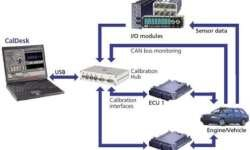Today, signals, i.e., quantities that fluctuate over time with high frequency, have acquired a great amount of importance and are being used in many fields, especially communication. Digital signal processing involves converting digital data into signals, making its transfer easier and subsequently converting these signals back into the original form. A signal has many characteristics or domains such as time domain, spatial domain, frequency, wavelet domain, etc. Anyone among these can be used to process a respective signal. From among these, the engineer usually selects the one that best represents the characteristics of the signal concerned or, in other words, the one from which data can be obtained easily. To ascertain the required characteristic, the engineer may try out many among these properties. The use of signals has gone up especially with the use of computers. Computers can analyze and process only digital (discrete) data and cannot handle analog (continuous) data. Thus, conversion of the signal from analog to the digital form becomes necessary. The digital signal is exactly similar to the analog signal that it has been obtained from; some mathematical techniques such as the Nyquist-Shannon sampling theorem are used. Usually, after analysis or transformation, the output signal is…

## Encoding and modulation of digital signals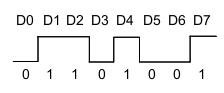We know that digital signals are stored as binary numbers in memory cells. Let’s see how these digital signals can be transferred, in other words – how they can be encoded into electrical signal shape. When the signal has to be sent, it has to be modulated. Pulse Code Modulation (PCM) Probably the simplest and the most common modulation method is pulse code modulation (PCM). In CPM, coding information is modulated as voltage levels. If the supply voltage is 5V, then binary”1″ is represented as +5V while 0 as 0V level. Only two voltage levels are used to modulate the signal. The signal can be sent in parallel (bus mode) or serial mode. In parallel mode whole (let’s say 8 bit) signal can be sent at once via 8 wires, while in serial mode, data has to be sampled in time. This transmission method is most popular in embedded systems. For instance, ADC sends converted data to DSP using CPM. CPM method is OK, but it has several drawbacks like the number of wires required for parallel buses. Still, probably the most dangerous problem is that transmission may experience skew problems when each data wire may have different time delays…

## DSP processors and their characteristics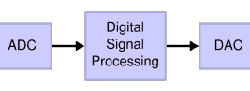The processing of the various signals is carried out through implements that are together called DSP hardware. This includes the hardware that is used for transmission of signals, multiple devices that are used to enhance or filter the signals, analog to digital and digital to analog converters, and other processing equipment such as computers. Among the hardware mentioned above, digital signal processors are the ones in which the actual processing occurs. Usually, the digital signal processors today have the following characteristics: They are equipped to handle real-time processing, i.e., they can give the optimal performance even when streaming data is being fed into them. The memories that are used to store programs are different from the ones used to store data. They do not provide hardware that supports multitasking. It can be used as a direct memory access device in supporting or host environments. They take analog signals as input, convert them into the digital form, process the signals, and then, Specific them back into the analogous form. They make use of the Direct Memory Access technique. The digital signal processors usually have architecture so as to optimise the following features:

## Digital Signal Processing – ApplicationsDigital signal processing is the technique used to analyze various digital signals and obtain information from the same. It is also used to transfer information from one place to another and involves conversion between analog and digital signals. It finds its application in various areas ranging from broadcasting to medicine. Let us have a look at some of the applications of the same. Biomedical Applications DSP is used extensively in the field of biomedicine. In it, the various signals generated by the different organs in the human body are measured to find information regarding the health of the same. For example, in the case of electrocardiograms, the electric signals generated by the heart are measured. Similarly, the activity of the brain is monitored by electroencephalograms. Automatic Control These days, many gadgets are available that can perform their tasks automatically. These devices contain various components that can take inputs depending on the surrounding conditions. These are conveyed to the device’s control unit, where they are processed, and the necessary action is taken. For example, a device like a thermostat increases its resistance in proportion to temperature. This can be used to stem the current in a machine whenever the temperature rises.

## Circular buffers in DSP processors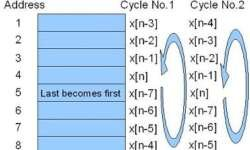I will not analyze what DSP processors are different from regular microcontrollers as many differences allow boosting performance in many specific tasks like filtering, FFT, etc. One thing is obvious that DSP processors have to perform mathematical calculations rapidly enough to get predictive results. The better result we want – the more processing power we need. We know that MCU is performing two main tasks: data manipulation and mathematical operations. But the fact is that it has to be done really fast. General-purpose microcontrollers aren’t optimized to perform these tasks effectively as microcontroller has to as much universal as possible to fit in many areas. In other words, flexibility reduces performance. DSP processors are more specialized microprocessors optimized for tas that they usually do – multiplication and addition. Let’s take the most common DSP routine FIR digital filter implementation. It takes several samples of signal x[] and produces output signal y[], modified by multiplying appropriate samples by coefficients an. y[n] = a0x[n]+a1x[n-1]+…+akx[n-k]

## Low frequency Butterworth and optimal Wiener ECG filtersRegular ad hoc filters don’t guarantee optimal signal filtering as there are no criteria that evaluate filter characteristics. Usually, filter parameters are calculated empirically, and the best results do filtering. To avoid such a shortage, there are optimal filters used where some criteria optimize parameters. Optimal filtering’s main idea is to give bigger weight coefficients to signal spectra parts where signal noise has less power, and true signal spectral components have bigger power. Let’s project a simple Butterworth filter used as a comparative filter to optimal Wiener DSP filter.Butterworth filter transfer characteristics: Where N indicates filter Tap number. I will skip the Butterworth filter description as the main idea is constructing an optimal Wiener filter. Butterworth filter characteristics are pretty plain: The main disadvantage of the Butterworth filter is that signal is distorted on filter output. If you want minimal signal distortions it is better to use an optimal Wiener filter. Filter chart looks as follows:

## Signal power and energy calculation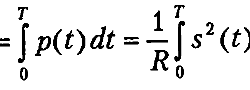The most common signal characteristics are energy and power. In signal theory, these terms require additional comments because they are different from what we are using in AC or DC systems. What are power and energy? When we connect the R resistor to voltage U, the resistor will dissipate some power equal to P=U2/R. During time T, the energy loss on this resistor will be E= TU2/R. Now let us say that we add some signal s() instead of DC voltage. In this case, the power will depend on time as the signal is time-dependent. The term is called instantaneous power: p(s)=s(t)2/R